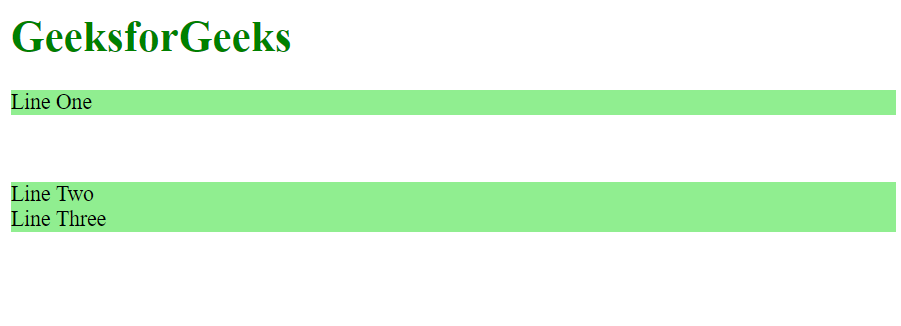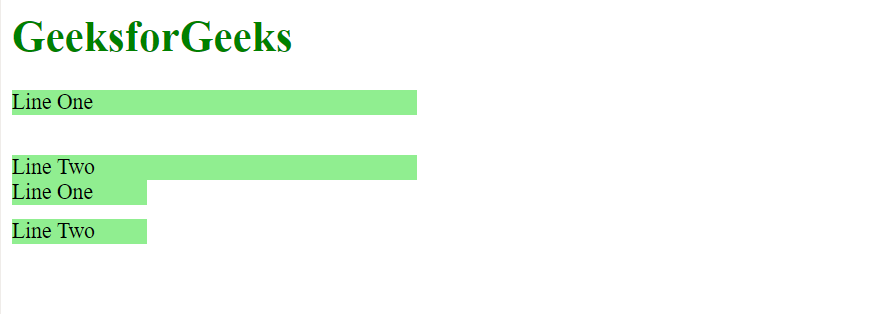# CSS margin-bottom Property

The CSS margin-bottom property is used to specify the amount of margin to be used on the bottom of an element. The margin can be set in terms of length or percentage.

Syntax:

`margin-bottom: <length> | <percentage> | auto`

Property values:

• Length: This value specifies the length of margin with a fixed value. This value can be positive, negative or zero.

Example:

## html

 `` `<``html``>` `<``head``>` `    ``<``title``>CSS margin-bottom` `    ``<``style``>` `        ``div{` `            ``background-color: lightgreen;` `        ``}` `    ``` `` `<``body``>` `    ``<``h1` `style="color: green">GeeksforGeeks` `    `  `    ``` `    ``<``div` `style="margin-bottom: 50px">Line One` `    `  `    ``` `    ``<``div` `style="margin-bottom: 0px">Line Two` `    `  `    ``<``div``>Line Three` `` `                    `

Output:• Percentage: This value specifies the amount of margin as a percentage relative to the width of the containing element.

Example:

## html

 `` `<``html``>` `<``head``>` `    ``<``title``>CSS margin-bottom` `    ``<``style``>` `        ``h1 {` `            ``color: green;` `        ``}` `        `  `        ``.larger {` `            ``width: 300px;` `            ``background-color: white;` `        ``}` `        `  `        ``.smaller {` `            ``width: 100px;` `            ``background-color: white;` `        ``}` `        `  `        ``div{` `            ``background-color: lightgreen;` `        ``}` `    ``` `` `<``body``>` `    ``<``h1``>GeeksforGeeks` `    `  `    ``` `    ``<``div` `class="larger">` `        ``<``div` `style="margin-bottom: 10%";>Line One` `        ``<``div``>Line Two` `    ``` `    `  `    ``` `    ``<``div` `class="smaller">` `        ``<``div` `style="margin-bottom: 10%;">Line One` `        ``<``div``>Line Two` `    ``` `` `                    `

Output:• auto: If the value of this property is set as “auto” then the browser automatically calculates a suitable value for the margin size.

Supported Browsers: The browser supported by margin-bottom property are listed below: